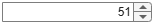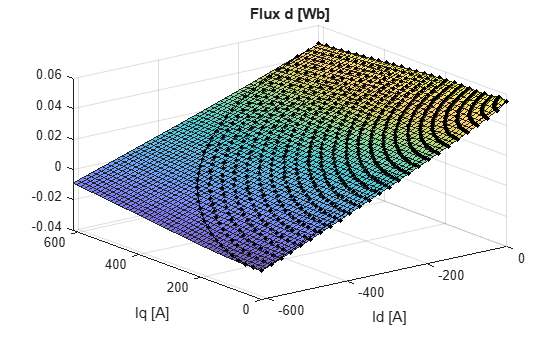# Generate Feed-Forward Flux Parameters

This example shows how to create lookup tables for an interior permanent magnet synchronous motor (PMSM) controller that characterizes the d-axis and q-axis flux as a function of d-axis and q-axis currents.

To generate the flux parameters for the Flux-Based PM Controller block, follow these workflow steps.

### Step 1: Load and Preprocess Data

Load and preprocess this nonlinear motor flux data from dynamometer testing or finite element analysis (FEA):

• d- and q- axis current

• d- and q- axis flux

• Electromagnetic motor torque

Import and preprocess the raw PMSM data from a `|xlsx|` file captured from a dynamometer or another CAE tool.

```fileName ="PMSM_150kW.xlsx"; opts = detectImportOptions(fileName); % read units if they are defined in the second row if opts.DataRange=="A3" opts.VariableUnitsRange = "A2"; end rawData = readtable(fileName, opts);```

```lambda_d = rawData.Psi_d; lambda_q = rawData.Psi_q; id = rawData.I_d; iq = rawData.I_q;```

### Step 2: Generate Evenly Spaced Data

The raw current and flux tables are recorded in a single column format. Convert them into evenly spaced data matrices using 2D interpolation.

Set the spacing for the table rows and columns.

```flux_d_size =51; flux_q_size =51;```

Use interpolation to get higher resolution.

```id_new = linspace(min(id),max(id),flux_d_size); iq_new = linspace(min(iq),max(iq),flux_q_size); currentBreakpoints = {id_new,iq_new};```

#### Visualize the Flux Surfaces

The d-axis flux, λd, is a function of d-axis current, Id, and q-axis current, Iq.

```lambda_d_new = fitlookupn(currentBreakpoints,[id,iq],lambda_d,ShowPlot=true); title("Flux d [Wb]"); xlabel("Id [A]") ylabel("Iq [A]");```The q-axis flux, λq, is a function of d-axis current, Id, and q-axis current, Iq.

```lambda_q_new = fitlookupn(currentBreakpoints,[id,iq],lambda_q,ShowPlot=true); title("Flux q [Wb]"); xlabel("Id [A]") ylabel("Iq [A]");```### Step 3: Set Block Parameters

Set these Flux-Based PM Controller block parameters to the values assigned in the script.

Vector of d-axis current breakpoints, id_index

`id_index=id_new;`

Vector of q-axis current breakpoints, iq_index

`iq_index=iq_new;`

Corresponding d-axis flux, lambda_d

`lambda_d=lambda_d_new;`

Corresponding q-axis flux, lambda_q

`lambda_q=lambda_q_new;`